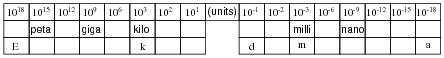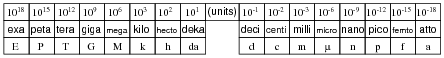# Learning Electronics

Learn to build electronic circuits

# Scientific notation and metric prefixes

### Question 1:

Write the following exponential expressions in expanded form:

 101 =

 102 =

 103 =

 104 =

 10-1 =

 10-2 =

 10-3 =

 10-4 =

 101 = 10

 102 = 100

 103 = 1000

 104 = 10000

 10-1 = 1 10

 10-2 = 1 100

 10-3 = 1 1000

 10-4 = 1 10000

Notes:
I have found that many students entering college (at least those entering a technical college in the United States) have a very limited understanding of exponents, especially negative exponents. You may find it necessary to review this concept, explaining what a negative exponent is and how it is defined.

### Question 2:

Evaluate the following arithmetic expressions, expressing the answers in expanded form:

 3.6 ×102 =

 1.53 ×10-4 =

 8.2 ×101 =

 6 ×10-3 =

Comment on the effect multiplication by a power of ten has on how a number is written.

 3.6 ×102 = 360

 1.53 ×10-4 = 0.000153

 8.2 ×101 = 82

 6 ×10-3 = 0.006

The effect that multiplication by a power of ten has on a number's written expression should be obvious: the decimal point gets shifted.

Notes:
The fact that multiplication by powers of ten simply shifts the decimal point is not a coincidence. Rather, it is a logical consequence of using a base-10 numeration system. If we all used binary for our daily enumeration, we would be using "powers of two" for scientific notation!

### Question 3:

Express the following numbers in scientific notation:

 0.00045 =

 23,000,000 =

 700,000,000,000 =

 0.00000000000000000001 =

 0.000098 =

 0.00045 = 4.5 ×10-4

 23,000,000 = 2.3 ×107

 700,000,000,000 = 7 ×1011

 0.00000000000000000001 = 1 ×10-20

 0.000098 = 9.8 ×10-5

Follow-up question: explain why scientific notation is used so frequently by scientists and engineers.

Notes:
The convenience in which scientific notation allows us to express and manipulate extremely large and small quantities is perhaps the most important reason why it is used by scientists and engineers.
Your students will be pleased to discover that their calculators can be used to easily convert between ëxpanded" (sometimes called fixed-point) and scientific notations.

### Question 4:

Metric prefixes are nothing more than ßhorthand" representations for certain powers of ten. Express the following quantities of mass (in units of grams) using metric prefixes rather than scientific notation, and complete the ïndex" of metric prefixes shown below:

 8.3 ×1018 g =

 3.91 ×1015 g =

 5.2 ×1012 g =

 9.3 ×109 g =

 6.7 ×106 g =

 6.8 ×103 g =

 4.5 ×102 g =

 8.11 ×101 g =

 2.1 ×10-1 g =

 1.9 ×10-2 g =

 9.32 ×10-3 g =

 1.58 ×10-6 g =

 8.80 ×10-9 g =

 6.9 ×10-12 g =

 7.2 ×10-15 g =

 4.1 ×10-18 g =8.3 ×1018 g = 8.3 Eg

 3.91 ×1015 g = 3.91 Pg

 5.2 ×1012 g = 5.2 Tg

 9.3 ×109 g = 9.3 Gg

 6.7 ×106 g = 6.7 Mg

 6.8 ×103 g = 6.8 kg

 4.5 ×102 g = 4.5 hg

 8.11 ×101 g = 8.11 dag

 2.1 ×10-1 g = 2.1 dg

 1.9 ×10-2 g = 1.9 cg

 9.32 ×10-3 g = 9.32 mg

 1.58 ×10-6 g = 1.58  mg

 8.80 ×10-9 g = 8.80 ng

 6.9 ×10-12 g = 6.9 pg

 7.2 ×10-15 g = 7.2 fg

 4.1 ×10-18 g = 4.1 agNotes:
Once students realize metric prefixes are nothing more than shorthand for certain powers of ten, they see that scientific and metric notations are really one and the same. The only difficult part of this is committing to memory all the different metric prefixes and their respective powers of ten. Be sure to mention that the power of ten that are multiples of three (103, 106, 10-12, etc.) are more commonly used than the other powers (h, da, d, and c).
In case anyone asks, the metric prefix for 101 (deka) is sometimes spelled deca. There seems to be no ßtandard" way to spell the name of this prefix.

### Question 5:

What is the difference between the following three values of mass (in kilograms)?

 4 ×102 kg

 4.5 ×102 kg

 4.500 ×102 kg

The difference between these figures is precision: the degree of uncertainty in the value expressed.

Notes:
It is important for students to understand that although a computer may see these three quantities as precisely equivalent, they are actually not. Human beings do not write extra zeros if they do not have to, and so these zeros serve to indicate extra certainty in the figure.